Next: A Lower Query Bound Up: Preliminaries Previous: Useful Definitions   Contents

### Quantum Oracle Models

In the quantum oracle model, we have an oracle that holds an N-bit input string. Our task is to determine the value of some fixed Boolean function of the oracle string, using as few oracle queries as possible. An oracle query is a question of the form: What is the ith bit of the oracle string?'' The quantum oracle model is a special case of Ambainis' more general quantum adversary model , which we describe below.

In the quantum adversary model, we run an algorithm against an oracle that contains a superposition of inputs. Let S be a subset of the possible inputs {0, 1}N. Algorithms in the quantum adversary model will work in the Hilbert space H = HA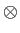HI, where HA =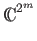is the Hilbert space of our m-qubit memory register, and HI =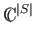is the Hilbert space spanned by basis vectors | x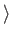corresponding to the elements of S. We think of HA as our algorithm space, and HI as our input space. The tensor product of two vector spaces A and B, denoted AB, is a new vector space spanned by all possible pairs (i, j) of basis vectors i from the first space and j from the second space. Thus H =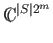.

We can represent the basis states of our algorithm space as | i, b, z, where i consists of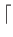logNbits, b is a single bit, z denotes all other bits our quantum algorithm requires. We define the oracle transformation O as the unitary operator that takes any eigenstate | i, b, z| xto | i, bxi, z| x. The firstlogNbits of the subspace defined by a particular input | xis the index i to the oracle bit xi that we are querying. O is a permutation matrix.

A quantum algorithm that performs T queries is just a sequence of unitary transformations

U0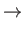OU1O...UT-1OUT,

where Ui is an arbitrary unitary transformation that does not depend on the oracle, and O is the oracle transformation. (Recall that unitary transformations are reversible and preserve the normalization of the state vector.)

The standard oracle query model is just an instance of the quantum adversary model where the input space is spanned by a single eigenstate | x. In this case a quantum algorithm starts with the state | 0| x, applies U0, O, U1,..., O, UT, and then measures the final state. The rightmost bit of the measured state of the algorithm space is the output of the algorithm on x. The algorithm computes f in the bounded error setting if for every input x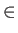{0, 1}N, the output is f (x) with some constant probability.

The measure of complexity in both the quantum oracle model and quantum adversary model is the number of oracle queries. It should be noted that querying the oracle is not always the most time consuming portion of an algorithm. For example, to factor an N bit integer that the oracle holds, we can determine the integer in N queries. However, we must then do 2N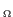(1) additional steps in the classical case, or N2logO(1)N additional steps in the quantum case to factor the number using the best known algorithms . Nonetheless we restrict our attention to the number of oracle queries required, as it is clearly a lower bound on the overall running time of the algorithm. Classical bounds for query complexity are well studied, making comparisons between quantum and classical oracle query complexity possible.Next: A Lower Query Bound Up: Preliminaries Previous: Useful Definitions   Contents
Matthew Hayward Lower Query Bounds in the Quantum Oracle Model GitHub Repository